Building Gopher services with Python

I've been working on a Gopher-based project in Python, because reasons. It was a refreshingly simple change from my usual web development work. Three evenings of work gave me a gopher server that presents all the content from a much more complex website.

Pituophis, a Python module for writing both Gopher servers and clients. Pituophis's default "handler" function acts as a basic Gopher server, publishing items in a specific directory to Gopherspace and uses an optional user-defined alternate handler function that responds when a missing file is requested. You can also replace the default handler and build your own Gopher application from the ground up.

One limitation of Pituophis compared to a web framework is the lack of a routing engine. That's easy enough to work around with a list of regular expressions and "controller" functions:

if request.path in ('', '/'):
return homePage(request)

paths = [
(re.compile('/image/([\w-]+)\.(gif|jpg|png)\$', re.IGNORECASE), sendImage),
(re.compile('/([a-z]{2})\$'), statePage),
(re.compile('/([a-z]{2})/([\w-]+)\$'), cityPage),
]

for pat, func in paths:
m = pat.match(request.path)
if m:
return func(request, m)

MiniZinc cryptarithms improved

Previously, I posted an experiment in solving cryptarithms with Python and MiniZinc. I've just put an improved version on GitHub, since I figured out how to generalize the problem somewhat--Python now just converts your problem into a dictionary and feeds that to an existing script that doesn't have to change. This new model still has some rough edges--artifacts of the learning process--but it works well enough.

Cryptarithms with MiniZinc, Python, and pymzn

A couple of entries in the AMS Page a Day Calendar so far have been cryptarithms. I most recently encountered these in the Coursera course on ("Basic Modeling for Discrete Optimization")](https://www.coursera.org/learn/basic-modeling), which uses them as an early example to teach MiniZinc. It's fairly easy to adapt existing code to solve this problem:

ZEROES + ONES = BINARY:

%From the AMS page-a-day calendar
var 0..9: Z;
var 0..9: E;
var 0..9: R;
var 0..9: O;
var 0..9: S;
var 0..9: N;
var 0..9: B;
var 0..9: I;
var 0..9: A;
var 0..9: Y;

constraint Z != 0;
constraint O != 0;
constraint B != 0;

constraint
100000 * Z + 10000 * E + 1000 * R + 100 * O + 10 * E + S
+                          1000 * O + 100 * N + 10 * E + S
= 100000 * B + 10000 * I + 1000 * N + 100 * A + 10 * R + Y;

include "alldifferent.mzn";
constraint alldifferent([Z,E,R,O,S,N,B,I,A,Y]);

%solve maximize (10000 * V + 1000 * E + 100 * R + 10 * S + E);

It's probably fairly easy to make a MiniZinc script that reads the problem from a data file instead of having it hardcoded, but I'm not that good with MiniZinc (I haven't finished the course). But this does seem like a good excuse to tinker with pymzn, a Python interface to MiniZinc. Here's my Python script to build a MiniZinc cryptarithm-solver script, run it, and return the results:

import io
import json
import re

import pymzn

def cryptarithm(problem):
mznscript = io.StringIO('')
prob = problem.replace(' ', '')
(facs, solution) = prob.split('=')
facs = facs.split('+')

#Set of all unique letters in the problem
letters = set(re.sub('[+=\s]', '', prob))
#All letters at the beginning of a "number"
initials = set([fac for fac in facs]+[solution])

#declare all variables.
#First letters != 0.
print(*[f'var 1..9: {l};' for l in initials], sep="\n", file=mznscript)
print(*[f'var 0..9: {l};' for l in letters.difference(initials)], sep="\n", end="\n\n", file=mznscript)

#Each letter stands for a different number.
print('include "alldifferent.mzn";', file=mznscript)
print(f'constraint alldifferent([{",".join(letters)}]);', file=mznscript)

print('\nconstraint', file=mznscript)

#This is ugly. Don't do this in actually-useful code.
print('    '+'\n  + '.join([' + '.join(f'{10**n} * {l}' for n,l in enumerate(reversed(tuple(fac)))) for fac in facs]), file=mznscript)
print('  = '+' + '.join([f'{10**n} * {l}' for n,l in enumerate(reversed(tuple(solution)))])+';', end="\n\n", file=mznscript)

print('solve satisfy;', file=mznscript)

mznscript.seek(0)

return (script, [dict(sorted(json.loads(sol).items(),  key=lambda x: problem.index(x)))
for sol
in pymzn.minizinc(script, output_mode='json')])

And some use examples:

>>> script, solutions = cryptarithm('ZEROES + ONES = BINARY')
>>> print(solutions)
[{'Z': 6, 'E': 9, 'R': 8, 'O': 3, 'S': 2, 'N': 1, 'B': 7, 'I': 0, 'A': 5, 'Y': 4}]

>>> script, solutions = cryptarithm('SEND+MORE=MONEY')
>>> print(solutions)
[{'S': 9, 'E': 5, 'N': 6, 'D': 7, 'M': 1, 'O': 0, 'R': 8, 'Y': 2}]

>>> script, solutions = cryptarithm('SO+MANY+MORE+MEN+SEEM+TO+SAY+THAT+THEY+MAY+SOON+TRY+TO+STAY+AT+HOME+SO+AS+TO+SEE+OR+HEAR+THE+SAME+ONE+MAN+TRY+TO+MEET+THE+TEAM+ON+THE+MOON+AS+HE+HAS+AT+THE+OTHER+TEN=TESTS')
>>> print(solutions)
[{'T': 9, 'A': 7, 'O': 1, 'M': 2, 'H': 5, 'S': 3, 'E': 0, 'N': 6, 'Y': 4, 'R': 8}]

>>> script, solution = cryptarithm('TWO + TWO = FOUR')
>>> print(solution)
[{'F': 1, 'T': 7, 'W': 3, 'R': 8, 'U': 6, 'O': 4}]

Kaprekar Process

The AMS Page a Day Calendar for January 17 discusses the Kaprekar Process:

D.R. Kaprekar discovered an interesting phenomenon that occurs when one takes a four-digit number, such that all four digits are not equal, and computes the difference between its decreasing and increasing rearrangements. He found that within seven iterations of this process you will always reach the number 6174, and this process became known as the Kaprekar Process.

-- Ellis, R., & Lewis, J. (2017). Investigations into the Kaprekar Process. Rose-Hulman Undergraduate Mathematics Journal, 3(2). https://scholar.rose-hulman.edu/rhumj/vol3/iss2/4

It's easy enough to do this calculation in Python

def kaprekar(x):
i = 0
xs = []
while True:
xs.append(x)
if x in (6174,0):
return (i, xs)
i+=1
while x < 1000: x = x * 10
x = list(str(x))
x1 = int(''.join(sorted(x)))
x2 = int(''.join(sorted(x, reverse=True)))
#print(''.join(x), x1, x2)
x = max([x1, x2]) - min([x1, x2])
>>> kaprekar(2021)
(3, [2021, 2088, 8532, 6174])
>>> kaprekar(4627)
(7, [4627, 5175, 5994, 5355, 1998, 8082, 8532, 6174])

We can also verify that at most seven iterations are required to reach 6174:

>>> ks = [kaprekar(x) for x in range(1,9999)]
>>> max([cnt for cnt, hist in ks])
7
>>> from collections import Counter
>>> Counter([x for x in ks]).most_common()
[(3, 2400), (7, 2184), (6, 1656), (5, 1518), (4, 1272), (2, 576), (1, 391), (0, 1)]

And finally, for the sake of having an image on this post:

>>> sns.set_theme(style="whitegrid")
>>> sns.countplot(x=[x for x in ks], color=(.3, .7, .3), alpha=.8, saturation=.3)

This visualization was the first draft of the last chart in this post. I added extra spacing between the bars to provide space for annotations; eventually I decided this was unnecessary.

#y-positions for the next graph. Add empty spaces (label='' and width=0) under each bar.
ypos = np.arange(chapterdata.shape*2 - 1, 0, -1)
widths = list(itertools.chain.from_iterable((x, 0) for x in chapterdata['new_vocabulary']))[:-1]
labels = list(itertools.chain.from_iterable((str(x), '') for x in chapterdata.index))[:-1]

#Don't need extra room after the first two chapters.
ypos = np.delete(ypos, (0,1))
del(widths)
del(widths)
del(labels)
del(labels)
MOST_COMMON_COUNT = 5

fig, ax = plt.subplots(figsize=(14, 40))
#plt.barh('new_vocabulary', chapterdata.index, data=chapterdata,orient='h', color=mycolor)

ax.barh(ypos, widths, .8, tick_label=labels, color=mycolor)
plt.ylim([0, ypos.max()+1])
plt.grid(axis='x')
xax = ax.get_xaxis()
xax.set_label_position('top')
plt.xlabel("Count of New Vocabulary")
ax.xaxis.tick_top()

for chapnum in range(1, 58):
chaplbl = str(chapnum)
#print(str(widths[labels.index(chaplbl)]))
plt.text(10, ypos[labels.index(chaplbl)], str(widths[labels.index(chaplbl)]),\
weight='bold', color='white', verticalalignment='center')
if chapnum >= 3:
newwords = ', '.join(['"%s" (%d)'%word for word in newvocab[chapnum].most_common(MOST_COMMON_COUNT)])
#print(newwords)
plt.text(10, ypos[labels.index(chaplbl)]-1.1, 'Major new vocabulary:', weight='bold',\
bbox=dict(facecolor='#ffffff99'))
plt.text(500, ypos[labels.index(chaplbl)]-1.1, newwords,\
bbox=dict(facecolor='#ffffff99'))

plt.show()

Here's one of the also-ran graphs I mentioned in my last post about Pickwick. They were part of my exploration of the data and didn't seem interesting enough to include in the last post.

The first shows the count of unique vocabulary per chapter, which isn't all that interesting without any context. It might work better as a stacked bar with unique vocabulary and total word count for each chapter.

#First make a DataFrame with chapter #s and word counts.
clengths = pd.DataFrame((
(x, len(chaptervocab[x-1])) for x in range(1,58)),
columns=['Chapter', 'Count of Unique Words'])
#Bar color for the rest of the plots.
mycolor="#3E000C"
Set the size of the plot.
plt.figure(figsize=(12, 20))
#plt.xlim(0,10000)
#Choose Seaborn display settings.
sns.set(style="whitegrid")
#Make a horizontal (orient='h') barplot.
sns.barplot('Count of Unique Words', 'Chapter',
data=clengths, orient='h', color=mycolor)

plt.show()
#I don't need this any more.
del(clengths)

Python, newspaper, and NLTK

I've been continuing my experimentation with NLTK, using the Python newspaper module. While newspaper seems mainly intended for making personalized Google News-like sites, it does some language processing to support this. Below, I continue where newspaper leaves off using NLTK and seaborn to explore an online text.

In :
import re # For some minor data cleaning

from IPython.core.display import display, HTML
import newspaper
import nltk
import matplotlib.pyplot as plt
import pandas as pd
import seaborn as sns

%matplotlib inline

I have several test sites in the cell below--sites are testy about allowing non-browser clients access and I've found I need to alternate while experimenting. Most of these sites work well, but newspaper often gives weird results from the Bing News search.

In :
#paper = newspaper.build('https://www.providencejournal.com/')
#paper = newspaper.build('https://www.bing.com/news/search?q=providence')
#paper = newspaper.build('https://www.guardian.co.uk')
paper = newspaper.build('https://www.vulture.com/')
len(paper.articles)
Out:
69

newspaper basics¶

Here I choose an article and download() and parse() it--which makes its data available. Then we can pull some basic details like the article's title, address, images, and text:

In :
#We *can* do this, but I want to make sure I have a relatively-long article to use.
a = paper.articles

#Via aldaily.com. Longer articles make more interesting graphs.
a = newspaper.Article('https://themillions.com/2020/03/on-pandemic-and-literature.html')
a.parse()

display(HTML("<h3>%s</h3>" % a.title))
print(a.url)

#display(HTML('<img src="%s"/>'%a.top_image))
print(a.top_image) # also a.images, a.movies
print(a.text[:500])

On Pandemic and Literature

https://themillions.com/2020/03/on-pandemic-and-literature.html
Less than a century after the Black Death descended into Europe and killed 75 million people—as much as 60 percent of the population (90% in some places) dead in the five years after 1347—an anonymous Alsatian engraver with the fantastic appellation of “Master of the Playing Cards” saw fit to depict St. Sebastian: the patron saint of plague victims. Making his name, literally, from the series of playing cards he produced at the moment when the pastime first became popular in Germany, the engrave

Our Article also gets a list of the authors of the text. I've found this tends to be the least accurate piece of newspaper's processing.

In :
a.authors
Out:
['Ed Simon',
'Ken Hines',
'Kirsty Logan',
'Patrick Brown',
'Emily St. John Mandel',
'Diksha Basu',
'Sonya Chung',
'Andrew Saikali']

NLP with newspaper¶

The .nlp() method gives us access to a summary of the text and a list of keywords. I haven't looked at the source closely enough to figure out how it's determining these, though the keywords are approximately the most common non-stopwords in the article.

In :
a.nlp()

print(a.summary)
display(HTML('<hr/>'))
print(a.keywords)
There has always been literature of pandemic because there have always been pandemics.
What marks the literature of plague, pestilence, and pandemic is a commitment to try and forge if not some sense of explanation, than at least a sense of meaning out of the raw experience of panic, horror, and despair.
Narrative is an attempt to stave off meaninglessness, and in the void of the pandemic, literature serves the purpose of trying, however desperately, to stop the bleeding.
Pandemic literature exists not just to analyze the reasons for the pestilence—that may not even be its primary purpose.
The necessity of literature in the aftermath of pandemic is movingly illustrated in Emily St. John Mandel’s novel Station Eleven.

['pandemic', 'disease', 'narrative', 'sense', 'black', 'writes', 'plague', 'death', 'literature', 'world']

NLP with NLTK¶

That's what Newspaper can do for us. But since I had nltk installed already (and Newspaper requires it even if I hadn't), I can take the this article's text and do some basic processing with it.

First I need to tokenize the text, breaking it into individual words and punctuation marks.

In :
a.tokens = nltk.word_tokenize(a.text)
print(a.tokens[:50])
['Less', 'than', 'a', 'century', 'after', 'the', 'Black', 'Death', 'descended', 'into', 'Europe', 'and', 'killed', '75', 'million', 'people—as', 'much', 'as', '60', 'percent', 'of', 'the', 'population', '(', '90', '%', 'in', 'some', 'places', ')', 'dead', 'in', 'the', 'five', 'years', 'after', '1347—an', 'anonymous', 'Alsatian', 'engraver', 'with', 'the', 'fantastic', 'appellation', 'of', '“', 'Master', 'of', 'the', 'Playing']

Next, guess each token's part of speech, using NLTK's "off-the-shelf" English tagger. This returns a list of 2-tuples (token, tag from the Penn Treebank tagset.

In :
a.pos_tags = nltk.pos_tag(a.tokens)
a.pos_tags[:15]
Out:
[('Less', 'JJR'),
('than', 'IN'),
('a', 'DT'),
('century', 'NN'),
('after', 'IN'),
('the', 'DT'),
('Black', 'NNP'),
('Death', 'NNP'),
('descended', 'VBD'),
('into', 'IN'),
('Europe', 'NNP'),
('and', 'CC'),
('killed', 'VBD'),
('75', 'CD'),
('million', 'CD')]

The Treebank tagset isn't particularly intuitive, especially if your last contact with English grammar was in middle school. Here's the help text for a few of the less-obvious tags above.

In :
for pos in ['NNS', 'NNP', 'IN', 'DT', 'JJ']:
nltk.help.upenn_tagset(pos)
NNS: noun, common, plural
undergraduates scotches bric-a-brac products bodyguards facets coasts
divestitures storehouses designs clubs fragrances averages
subjectivists apprehensions muses factory-jobs ...
NNP: noun, proper, singular
Motown Venneboerger Czestochwa Ranzer Conchita Trumplane Christos
Oceanside Escobar Kreisler Sawyer Cougar Yvette Ervin ODI Darryl CTCA
Shannon A.K.C. Meltex Liverpool ...
IN: preposition or conjunction, subordinating
astride among uppon whether out inside pro despite on by throughout
below within for towards near behind atop around if like until below
next into if beside ...
DT: determiner
all an another any both del each either every half la many much nary
neither no some such that the them these this those
third ill-mannered pre-war regrettable oiled calamitous first separable
ectoplasmic battery-powered participatory fourth still-to-be-named
multilingual multi-disciplinary ...

I'll also tag the article using the "Universal" tagset--it has fewer tags, which makes for a simpler visualization later on.

In :
a.upos_tags = nltk.pos_tag(a.tokens, tagset="universal")
a.upos_tags[:15]
Out:
('a', 'DET'),
('century', 'NOUN'),
('the', 'DET'),
('Black', 'NOUN'),
('Death', 'NOUN'),
('descended', 'VERB'),
('Europe', 'NOUN'),
('and', 'CONJ'),
('killed', 'VERB'),
('75', 'NUM'),
('million', 'NUM')]

We can also have NLTK calculate a frequency distribution of the words in our article--here I'll use it to show the most common 10 tokens, most of which you probably could have guessed:

In :
a.word_freqs = nltk.FreqDist(word.lower() for word in a.tokens)
a.word_freqs.most_common(10)
Out:
[('the', 310),
(',', 262),
('of', 209),
('and', 112),
('.', 112),
('a', 88),
('to', 85),
('that', 80),
('’', 70),
('in', 61)]

Visualization¶

NLTK's FreqDist can also generate plots. Not great plots. Here's an example.

In :
plt.figure(figsize=(12, 8))
a.word_freqs.plot(25)
plt.show()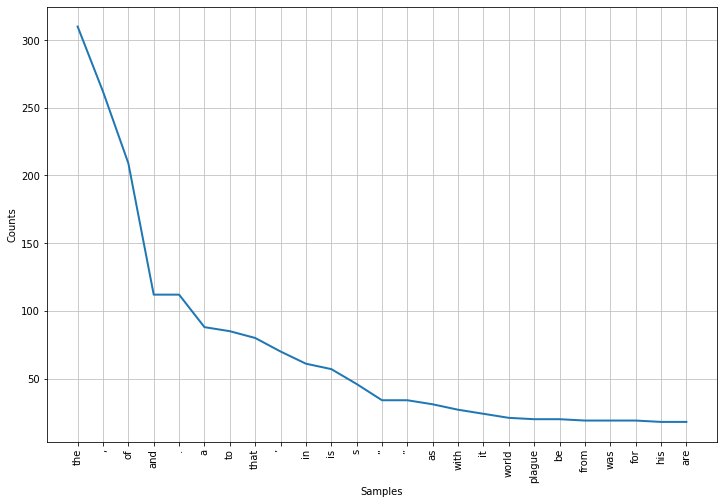Line graphs usually make me think "time series". This should probably be a bar plot, and we can do that. Start by translating our FreqDist object's data to a pandas DataFrame:

In :
wfdf = pd.DataFrame(a.word_freqs.items(), columns=['token', 'frequency'])
Out:
token frequency
0 less 2
1 than 13
2 a 88
3 century 4
4 after 9

We can now generate a Seaborn barplot of the token frequency data, which is largely unsurprising.

In :
mycolor="#3E000C" #"#9A1C42"
plt.figure(figsize=(12, 8))
sns.set(style="whitegrid")
sns.barplot('frequency', 'token', data=wfdf.sort_values(by='frequency', ascending=False)[:25], color=mycolor)
plt.show()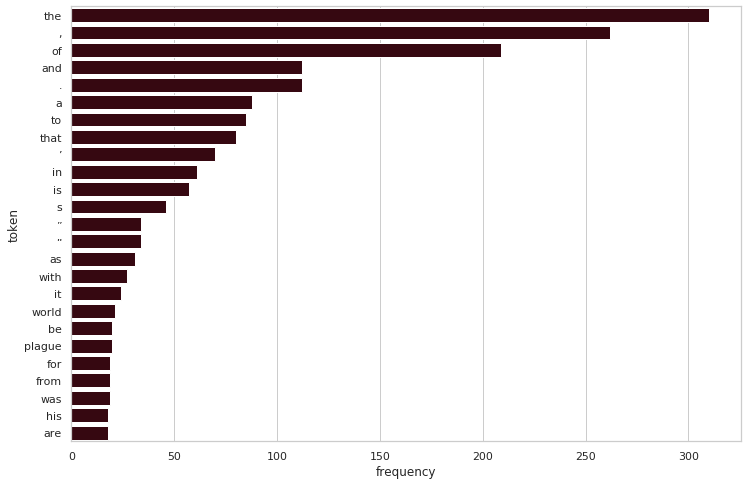We can make the result (arguably) more interesting by removing stopwords--very common words that don't affect the meaning of the text--from the frequency list. Here we get the stopwords for English.

In :
sw = nltk.corpus.stopwords.words('english')
print(sw)
['i', 'me', 'my', 'myself', 'we', 'our', 'ours', 'ourselves', 'you', "you're", "you've", "you'll", "you'd", 'your', 'yours', 'yourself', 'yourselves', 'he', 'him', 'his', 'himself', 'she', "she's", 'her', 'hers', 'herself', 'it', "it's", 'its', 'itself', 'they', 'them', 'their', 'theirs', 'themselves', 'what', 'which', 'who', 'whom', 'this', 'that', "that'll", 'these', 'those', 'am', 'is', 'are', 'was', 'were', 'be', 'been', 'being', 'have', 'has', 'had', 'having', 'do', 'does', 'did', 'doing', 'a', 'an', 'the', 'and', 'but', 'if', 'or', 'because', 'as', 'until', 'while', 'of', 'at', 'by', 'for', 'with', 'about', 'against', 'between', 'into', 'through', 'during', 'before', 'after', 'above', 'below', 'to', 'from', 'up', 'down', 'in', 'out', 'on', 'off', 'over', 'under', 'again', 'further', 'then', 'once', 'here', 'there', 'when', 'where', 'why', 'how', 'all', 'any', 'both', 'each', 'few', 'more', 'most', 'other', 'some', 'such', 'no', 'nor', 'not', 'only', 'own', 'same', 'so', 'than', 'too', 'very', 's', 't', 'can', 'will', 'just', 'don', "don't", 'should', "should've", 'now', 'd', 'll', 'm', 'o', 're', 've', 'y', 'ain', 'aren', "aren't", 'couldn', "couldn't", 'didn', "didn't", 'doesn', "doesn't", 'hadn', "hadn't", 'hasn', "hasn't", 'haven', "haven't", 'isn', "isn't", 'ma', 'mightn', "mightn't", 'mustn', "mustn't", 'needn', "needn't", 'shan', "shan't", 'shouldn', "shouldn't", 'wasn', "wasn't", 'weren', "weren't", 'won', "won't", 'wouldn', "wouldn't"]

And next, remove stopwords (and punctuation) from our word frequency list, and create a new DataFrame and a new graph.

In :
# '\W' matches one "non-word character", i.e., not a letter, number or underscore.
wf2 = [word for word in a.word_freqs.items() if word not in sw and not re.match('\W', word)]
wf2df = pd.DataFrame(wf2, columns=['token', 'frequency'])

plt.figure(figsize=(12, 8))
sns.barplot('frequency', 'token', data=wf2df.sort_values(by='frequency', ascending=False)[:25], color=mycolor)
plt.show()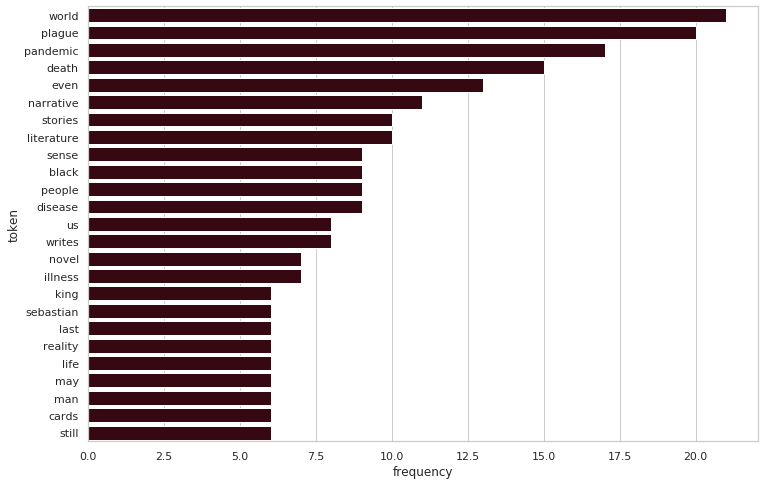This tells us more about the article. But what about the part-of-speech tags I generated earlier? Here's a function that will take those lists and generate a graph from them:

In :
def posFreqGraph(tags):
posfreqs = nltk.FreqDist(word for word in tags)
posfdf = pd.DataFrame(posfreqs.items(), columns=['pos', 'frequency'])
plt.figure(figsize=(12, 8))
sns.barplot('frequency', 'pos', data=posfdf.sort_values(by='frequency', ascending=False), color=mycolor)
plt.show()

First, the graph of Penn Treebank tags. It's crowded--which shows us the richness of this tagset--but still readable. (Here's a complete list of these tags with meanings.)

In :
posFreqGraph(a.pos_tags)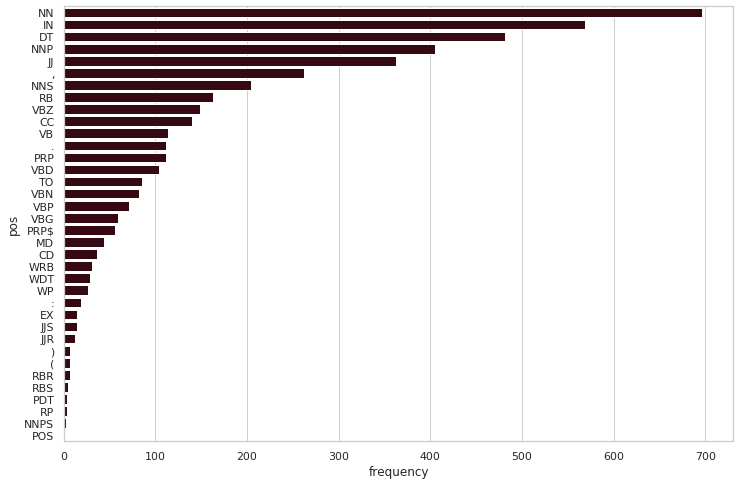Here's the same visual built from the universal tagset data.

In :
posFreqGraph(a.upos_tags)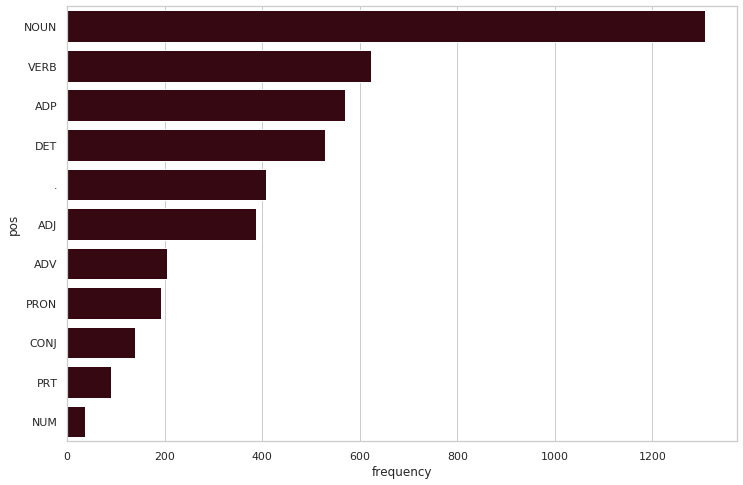Closing

I think that's a good start. Newspaper makes it easy to load web pages and get just the important text, which we can feed into NLTK for some analysis. Here I did only the very basics of an analysis with NLTK; I plan to experiment more over the next few weeks.

Simple Text Collation with Python

Although in the study of manuscript culture one of the characteristic activities is to align parallel parts of a work–and this is a common definition of collation–I speak of collation in the more narrow sense of identifying differences between texts (i.e., after passages are already “aligned”). There are three methods to collate texts: 1) read two texts side by side and note the differences, 2) compare printed page images (by allowing your eyes to merge two page images, often with a device especially for that purpose); 3) transcribe and compare transcriptions with aid of a computer.

Last semester I taught an introductory programming course to non-Computer Science graduate students at URI. My curriculum focused mostly on the Python data science toolset of Jupyter, pandas, and numpy, and using these tools to analyze the students' own datasets.

One student, an English Ph.D. candidate, asked for an alternative involving natural language processing tasks: performing a collation of two editions of a novel released about a decade apart. This kind of work was new to me, but seemed like a simple enough task for a beginning programmer to handle.

My student had both editions of the book as PDFs (scanned from physical books with embedded OCRed text. We explored two modules for extracting the text:

PyPDF2 was our first try. Its getPage() method didn't include whitespace in its output, giving each page's text as a single long word, probably as a result of the PDF's internal formatting, as suggested by Ned Batchelder on StackOverflow. I suspect it would be simple enough to read each word and paste them together as needed, but it was easier to find another solution for PDF reading.

PyMuPDF just worked, at least well enough for this use. It added unnecessary newlines, which would have been a problem if we were interested in paragraph breaks but wasn't an issue here. It also failed with one file's dropcaps, which was probably more an OCR/encoding issue. Here's an example of use (output on the right; the file 01.01_Why_Model.pdf is one of the readings for Scott Page's Model Thinking course on Coursera):

import fitz
pdf = fitz.open('01.01_Why_Model.pdf')
text = ''for page in pdf: text += page.getText()

Text comparison with difflib

It took me an embarrassing amount of time before I realized the tool we needed here was diff. Python's difflib was the ideal solutions. It has a few basic options that easily produce machine-readable (like the command-line app) or HTML table output, but can also produce more complex output with a little effort. Its HtmlDiff tool worked perfectly for this.

The image to the right shows difflib's output from this code in a Jupyter window:

import difflib
from nltk import word_tokenize
from IPython.display import display, HTML

str1="This is my short string"
str2="This is another not long string"

words1 = word_tokenize(str1)
words2 = word_tokenize(str2)

hd = difflib.HtmlDiff()

HTML(hd.make_table(words1, words2))

Two other HtmlDiff options (to display only differences in context , and to limit the number of lines of context) were ideal for this case--we don't need to show the entire book just to print a relative handful of differences. For example, the following will only show changes with three words of context around each:

hd.make_table(context=True, numlines=3)

Closing

Once the difflib's HTML was output, the rest of the student's work on this project was reading through the table, identifying individual changes as 'substantive' or 'accidental', and tabulating them. But there's more we could do with Python to simplify this or enrich the final output, for example:

• Identify changes where a single punctuation mark was changed to another--many of these were probably either typos or OCR errors.
• Do part-of-speech tagging on the books' text and include this data in the output--did the author systematically remove adjectives and adverbs, or is there some other trend?